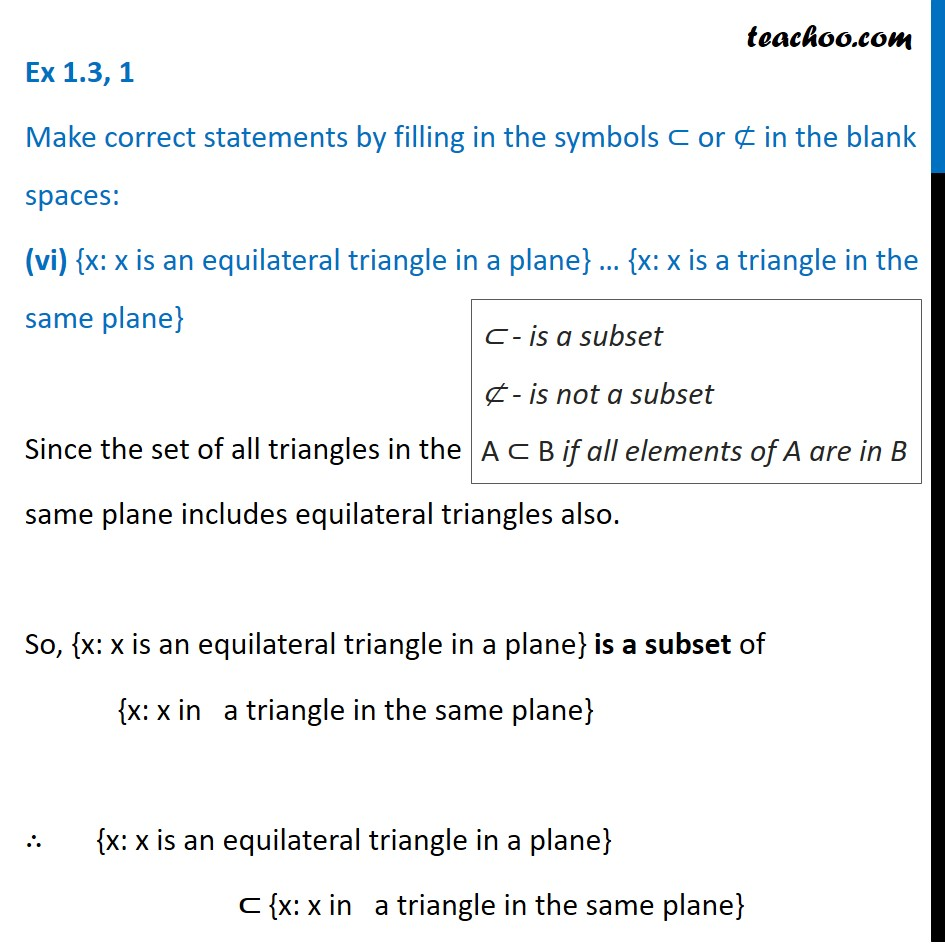Ex 1.3

Chapter 1 Class 11 Sets
Serial order wiseLearn in your speed, with individual attention - Teachoo Maths 1-on-1 Class

### Transcript

Ex 1.3, 1 Make correct statements by filling in the symbols ⊂ or ⊄ in the blank spaces: (vi) {x: x is an equilateral triangle in a plane} … {x: x is a triangle in the same plane} Since the set of all triangles in the same plane includes equilateral triangles also. So, {x: x is an equilateral triangle in a plane} is a subset of {x: x in a triangle in the same plane} ∴ {x: x is an equilateral triangle in a plane} ⊂ {x: x in a triangle in the same plane} ⊂ - is a subset ⊄ - is not a subset A ⊂ B if all elements of A are in B